nextnano.com  Tool: nextnano++  Tool: nextnano³  Download | Search | Copyright | Publications  * password protected nextnano³ softwareGlossary

# 3D Nano Device Simulator

## Glossary

last updated: 03-02-22

• ## Boundary conditions for the Poisson equation

Dirichlet boundary conditions specify the potential at the boundaries. The solution of the Poisson equation is then unique.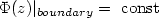Neumann boundary conditions specify the normal component of the electric field at the boundaries (i.e. the normal derivativ of Phi is specified on the surfaces). The solution of the Poisson equation is then unique apart from a constant.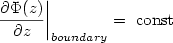By specifying both Dirichlet and Neumann boundary conditions, the system would be over-determined.
More details on boundary conditions can be found here.

• ## Calculation of the density

• purely classical calculation
• purely quantum mechanical calculation
• mixed classical/quantum mechanical calculation (only localised states are considered for quantum mechanical density)
• mixed classical/quantum mechanical calculation (in quantum regions the density is calculated purely quantum mechanically)

More details on the calculation of the density can be found here.

• ## Current continuity equations for electron and holes

 The continuity equation for electrons and holes: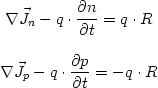More details...

• ## Current relations for electrons and holes

 The current relations for electrons and holes:More details...

• ## Eigenvalue problem on inhomogeneous grid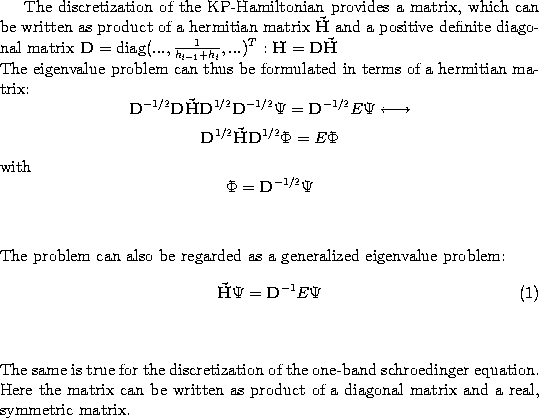• ## Generation of the grid

The parser program is mapping the geometry of the device onto discrete grid points to which a material is associated with. The points of the simulation grid are lying in between these material grid points. This approach is necessary because the set-up of the systems of differential equations is done via box integration. In 3D, each box consists of eight smaller boxes (octants) and each octant can uniquely be associated with one material grid point. The interfaces at material transitions are hence lying on the simulation grid points and therefore in the middle between the material grid points. The grid specifications in the input file are thus referring to the material grid points. This has to be considered on data entry.

• ## Method of Brillouin zone integration

1D simulation

Evaluating the density integration
The program provides three options for the calculation of the density when performing a 1D k.p calculation. For this, only k values up to a maximum value of k have to be considered. All values that are larger are lying energetically very far away from the Fermi level so that their contributions to the density can be neglected.
The option will be specified in the input file under the keywords $quantum-model-electrons/$quantum-model-holes:
Specifier method-of-brillouin-zone-integration = ...

= special-axis
In this case the Hamiltonian must be isotropic in the (kx, ky) plane, i.e. E(kx, ky) = E(k||) where k||² = kx² + ky². Then the integration of the density can be reduced to a 1D integral. This is only allowed for wurtzite in the  growth direction. In all other cases the program switches to simple-integration.

= simple-integration
The 2D Brillouin zone will be discretized and the density will be calculated by the summation over all k points.
For zinc blende with {001} quantization direction only the irreducible part of the Brillouin zone is being discretized (up to maximum values of k), correspong to about ~1/8 of the total number of k points. In all other cases the whole Brillouin zone is being discretized (up to maximum values of k).

= gen-dos
The evaluation of the density is done by integration over the density of states (DOS).
For zinc blende with {001} quantization direction only the irreducible part of the Brillouin zone is being discretized (up to maximum values of k), correspong to about ~1/8 of the total number of k points. In all other cases the whole Brillouin zone is being discretized (up to maximum values of k).

The number of discretization points has to be specified under the specifier 'num-kp-parallel' and refers to the discretization of the whole Brillouin zone up to the maximum value of k. For the special cases special-axis and zinc blende in {001} direction, the program is calculating the number of actually needed points.

More details can be found here and here and here:
  $quantum-model-electrons$quantum-model-holes

2D simulation

Here, only
 = special-axis
and
 = gen-dos
are allowed. simple-integration does not make sense as the k space is only one-dimensional (kz along a line) in a 2D k.p calculation and thus it would be equivalent to special-axis.

3D simulation

Not applicable. This does not make sense for a 3D simulation.

• ## Poisson equation

 The Poisson equation: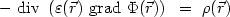epsilon is the dielectric constant varying over space.
Phi is the electric potential.
rho is the electric charge density (consists of electrons, holes, ionized donors and acceptors, piezo- and pyroelectric polarization charges).

Linear Poisson equation:
- if the charge density is constant, i.e. independent of the potential (e.g. piezo and pyro charges)

Nonlinear Poisson equation:
- charge density depends on potential

• ## Quasi-Fermi level

So-called "quasi-Fermi levels"  which are different for electrons (EF,n) and holes (EF,p) are used to describe nonequilibrium carrier concentrations.
In equilibrium the quasi-Fermi levels are constant and have the same value for both electrons and holes (EF,n = EF,p = 0 eV).
The current is proportional to the mobility and the gradient of the quasi-Fermi level EF.

• ## Separation model: Quantum and continuum states

Here are some technical details on states in quantum regions, namely the separation of classical and quantum mechanical states.

In quantum regions the density is determined by quantized wave functions.

The density there is calculated as follows:
- The quantized states are taken up to a certain energy (called separation energy).
- Above the separation energy the density is calculated by using the Thomas-Fermi approximation.

The separation energy can be determined by three different methods according to the specification in the input file:
(specifier 'separation-model = ...'  in $quantum-model-electrons/$quantum-model-electrons).

= eigenvalue:
For option 'eigenvalue'  (internally stored as 'eigenvalue') the specified number of eigenvalues (number-of-eigenvalues-per-band) is calculated and the topmost eigenvalue at the same time is the separation energy.
All eigenvalues up to number-of-eigenvalues-per-band are considered for the quantum mechanical density.
Above (below) topmost eigenvalue electrons (holes) are considered to be classical.
=> Separation energy = energy of topmost eigenvalue

= energy:
For option 'energy' (internally stored as 'max_energy') a fixed energy is provided by the input file ('maximum-energy-for-eigenstates') in units of [eV] which is given relative to the band edge extremum.
It has the following meaning:
For electrons take the minimum conduction band edge energy throughout the whole quantum region and let the separation energy be this minimum plus the fixed energy from the input file that does not change during the calculation.
(For holes: maximum valence band edge energy minus fixed energy)
All eigenstates up to this value are considered in quantum mechanical calculation.
Above (below) this value electrons (holes) are considered to be classical.
=> Electrons: Separation energy = lowest energy point in this quantum cluster + maximum-energy-for-eigenstates      Holes:   Separation energy = highest energy point in this quantum cluster - maximum-energy-for-eigenstates

= edge-model:
For option 'edge-model' (internally stored as 'edge_model')  the specified number of eigenvalues (number-of-eigenvalues-per-band) is calculated and the separation energy is determined by the following model:
- For electrons take the conduction band minimum energy of all boundary points of the quantum region.
(In 1D there are only two boundary points.)
(For holes: maximum valence band energy)
- All quantum states with energies below that level are certainly fully quantized. (for holes: above)
- So the highest eigenvalue that is just below this level is taken to be the separation energy.
Note that if all eigenvalues are below that level the highest it taken as the separation energy. (for holes: lowest)
Here, the separation energy is set to consider only localized states for the density, delocalized states are not considered.
Attention: This could lead to the situation in which no quantized states are considered if the choice of the quantum region is taken in an infavorable way. Thus one always has to check the position of the band edges and the eigenstates afterwards.
SeparationEnergyEdgeV = highest eigenvalue that is below minimum of conduction band edges on left and right boundary (1D) (for electrons)
at boundaries (2D/3D) (for electrons)
(for holes: lowest ... maximum of valence band edges)

More details for the separation model can be found here.

 If you need support, or input files that are not included in the installation folder, or if you have suggestions on how we can improve our website or tutorials, please submit a Support Ticket.